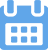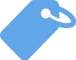# APY Meaning: Here’s What It Means and How To Use It

•Kevin
•March 1, 2022
•In this article, you’ll understand more about the meaning of APY through definitions, background information, explanations, examples, and more.### Your writing, at its best

Compose bold, clear, mistake-free, writing with Grammarly's AI-powered writing assistant

Abbreviations are useful ways of shortening a word, a group of words, or even a phrase. Typically, an abbreviation can be defined using the words that it represents. Sometimes, though, it’s necessary to take a closer look for a complete understanding.

It’s also common for abbreviations to have multiple meanings, so context is needed to fully comprehend the speaker or writer’s intention.

## What Does APY Mean?

APY is an abbreviation that means annual percentage yield. APY is essentially the amount of money or interest you earn on a bank account over one year. It is similar to a period rate for a specific period or the annual interest rate.

To fully appreciate the meaning of APY, it’s important to look at each word individually.

• Annual is an adjective that describes when something occurs once every year.
• Percentage is a noun that is any portion or share relative to the whole amount or unit.
• Yield is a verb that means produce or provide or a noun that refers to the full amount of an industrial product.

Using these definitions, it’s easy to understand that APY or annual percentage yield is the portion produced from the whole amount invested over a year. You can easily calculate APY on an account with the right formula.

## What Is the Origin of APY?

For United States’ financial institutions, APY is calculated and regulated by the FDIC Truth of Savings Act of 1991. These regulations are monitored and updated by Congress through laws and amendments. The APY calculated tells investors about the annual rate of interest an account has produced.

APY calculations are similar to other interest rate calculations. We can trace these calculations back to the work of Jacob Bernoulli in 1683, the first to discover “e.” This “e” is not to be confused with Euler’s number in other mathematical formulas. The basis for our calculations of APY is just like Bernoulli’s.

## How Can We Use APY?

An annual percentage yield is the amount of interest on a bank account in a year. An APY is similar to an interest rate, but it doesn’t include compounding interest. Some examples of accounts that create APY include:

• Savings accounts
• Cash management accounts
• CD accounts
• Checking accounts
• Money market accounts

### Calculating APY

APY is calculated using a formula, and you can calculate it manually if you know your account’s interest rate. The formula for APY is:

APY = (1 + r/n) ^ n -1

In the formula, the variables are defined as:

r = interest rate

n = number of compounding periods (when interest is compounding monthly, n would be 12)

If you have questions about your APY, your bank or credit union can provide you with the interest calculations. Some financial institutions may charge fees for calculating your APY. If this is true, you can instead use an online compound interest calculator.

### Comparing Accounts With APY

A high-yield APY is more desirable because the money you deposit in your interest-bearing account grows faster. The higher your savings account’s APY, the better. You’ll want to seek out high-yield savings accounts.

When you’re comparing deposit accounts, consider the compounding interest of online savings accounts and consider the compounding frequency offered by the online bank. The national average is 0.06%, and it should be considered when looking at a new account.

You’ll also want to know if your account is a variable rate account. The Federal Reserve is responsible for raising and lowering the interest rate.

Similarly, the interest rate of student loans is determined by Congress.

An APY is similar to an APR, which is an annual percentage rate. APR is useful when calculating the costs you can incur on a credit card, mortgage, auto loans, or other personal loans.

## What Are Synonyms for APY?

Synonyms have the same meaning as a subject word. When it comes to an abbreviation, you want to use a synonym for the group of words it represents. Here are synonyms for APY:

• Interest
• Bank rate
• Lending rate
• Prime rate
• Rate of interest
• Price of money

Some related terms to APY include:

• Annual Percentage Rate
• Annual Interest Rate

## What Are Antonyms for APY?

As with the synonyms of an abbreviation, it can be difficult to find antonyms for an abbreviation. An antonym has the opposite meaning of a subject word or phrase. APY does not have any known antonyms.

## Examples of How To Use APY

Example sentences are the easiest way to learn how to properly use a word, term, or abbreviation in context. Here are a few examples of how to use APY in a sentence:

• The account’s APY is calculated using a fixed rate.
• The APY uses the effective annual rate.
• We should compare the APY on the two investments.
• The APY was lower on the higher principal amount because of the lower compounding frequency of the interest.
• I’ve been monitoring the APY on this account for the last several years.

## The Last Word

Abbreviations can be used with confidence when you take the time to learn what they mean and the definition of the word or words they represent. Perhaps now that you know more about the meaning of APY, you’ll be able to use it and understand it in the real world.